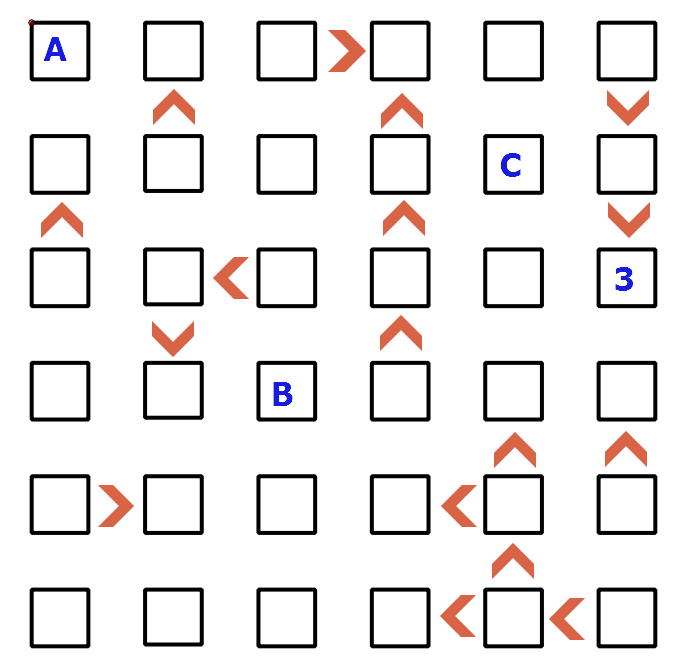# Classic Futoshiki

Logic Level 5Above shows a 6 by 6 square grid. Your objective is to place the numbers 1 to 6 such that each row and column contain each of the digits 1 to 6. The inequality constraints are specified between some of the squares to indicate one must be higher or lower than its neighbor. If $A,B,C$ indicate the numbers in the square boxes as shown above, find the value of $A\times B\times C$.

×